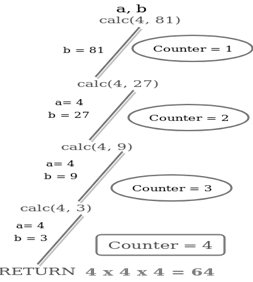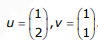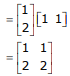# GATE Solved Paper 2017-19 - GATE 2018

• A

Schema I• B

Schema II• C

Schema III• D

Schema IV• Option : B
• Explanation :
In Schema II : Registration (rollno, courseid,
email)
Non-trivial functional dependencies:
{ rollno, courseid → email
email → rollno }
candidate keys
{rollno, courseid,}
email courseid}
Given relation is in 3NF but not in BCNF.

int counter = 0;
int calc(int a, int b) {
int c;
counter++;
if (b == 3)
return (a * a * a);
&emp;else {
c = calc(a, b / 3);
return (c * c * c);
}
}
int main() {
calc(4, 81);
printf("%d", counter);
}
The output of this program is ________ .

Note – Numerical Type question.

• A

5• B

4• C

3• D

None of these• Option : B
• Explanation :The largest eigenvalue of A is ________.

Note – Numerical Type question

• A

4• B

2• C

5• D

3• Option : D
• Explanation :A = UVT(1 - λ)(2 - λ) - 2 = 0
λ2 - 3λ = 0
λ(λ - 3) = 0
λ = 0
or, λ = 3
The largest eigen value is 3.

• A

2• B

4• C

3• D

5• Option : A
• Explanation :
Given,
Number of processes (P) = 3
Number of resources (R) = 4

Since deadlock-free condition is:

R ≥ P(N − 1) + 1
Where R is total number of resources,
P is the number of processes, and
N is the max need for each resource.

4 ≥ 3(N − 1) + 1
3 ≥ 3(N − 1)
1 ≥ (N − 1)
N ≤ 2
Therefore, the largest value of K that will always avoid deadlock is 2.
Option (A) is correct.

• A

40• B

42• C

44• D

46• Option : B
• Explanation :
Given |G| = 84
Solutions:
By Lagrang’s theorem any subgroup size is a divisior of 84.
But a proper subgroup cannot have same size as group.
So largest divisor of 84, other than 84 is 42.
So, largest proper subgroup can have in size of 42.
Related Quiz.
GATE 2018